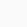# How to use norm ||a|| symbol in LaTeX?

SymbolNorm
Type of symbolMathematics
Package (requirement)No
ArgumentNo
Latex command\|..\|
Example\|a\| → ||a||

LaTeX has multiple methods of representing norm symbols. Some of these effective methods are discussed below.

\documentclass{article}
\newcommand{\mynorm}{ \left\| #1 \right\| }
\begin{document}
$\|u\|_2 = \sqrt{u{^2_1} + u{^2_2} + ... + u{^2_n}}$
$\frac{{\| \hat{x} - x\|}_2}{\|x\|}$
$\mynorm{\left( \sum_{n=1}^N P_n \right)}$
$\frac{d \|u\|_2}{du_1}$
\end{document}

Output :There is no default command for the Norm symbol, but it is in the physics package. In my opinion, it is best practice to use direct \norm{arg} commands rather than writing large syntax.

\documentclass{article}
\usepackage{physics}
\begin{document}
$\norm{\sum_{j=1}^{k}v_{j}}$
$\norm\big{\frac{v}{k}} \; \norm\Big{\frac{v}{k}} \; \norm\bigg{\frac{v}{k}} \; \norm\Bigg{\frac{v}{k}}$
\end{document}

Output :Scroll to Top